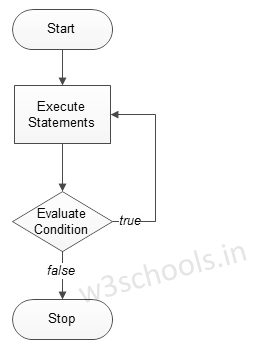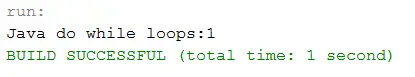## Java Programming Tutorial Index

Java do-while loops are very similar to the while loops, but it always executes the code block at least once and furthermore as long as the condition remains true. This loop is an exit-controlled loop.

The basic format of do-while loop statement is:

Syntax:

``````do
{
statement(s);

}while( condition );``````

Figure - Flowchart of the do-while loop:### Example of a Java Program to Demonstrate "do while" loop

Example:

``````public class Sample {

public static void main(String args[]) {
/* local variable Initialization */
int n = 1, times = 0;

/* do-while loops execution */
do {
System.out.println("Java do while loops:" + n);
n++;
} while (n <= times);
}
}``````

Program Output: